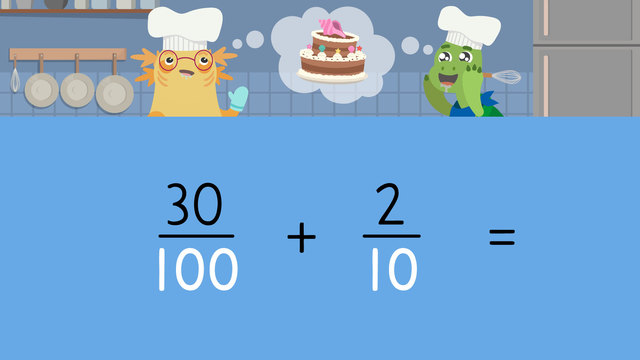Rating

Ø 5.0 / 1 ratings

The authorsTeam Digital
CCSS.MATH.CONTENT.4.NF.C.5

## Basics on the topicAdding Tenth and Hundredth

### In This Adding Tenths and Hundredths Video

Axel and Tank are baking a cake, with fractional parts of ingredients. They need to practice adding tenths and hundredths fractions so they know how much flour and sugar they’re using for their cake! At the end, you will find an adding tenths and hundredths fractions worksheet.

### Adding Fractions Tenths and Hundredths

When adding fractions with tenths and hundredths, first, set up the problem.Next, look at the denominator, the bottom number, of both fractions. Since they’re not the same, or like, we need to convert one fraction to have the same denominator as the other.To do this, we can multiply to fractions with the ten denominator by ten to make one hundred. When we do this, we also need to multiply the numerator, the top number, by ten as well.Now both denominators are one hundred, we can solve the problem!Adding Tenths and Hundredths - Summary

Remember, when adding tenths and hundredths fractions, make sure you have like denominators before solving the problem. The denominator of the sum will always remain the same, and you only need to add together the numerators.Below, you will find an adding tenths and hundredths worksheet, with adding tenths and hundredths questions.